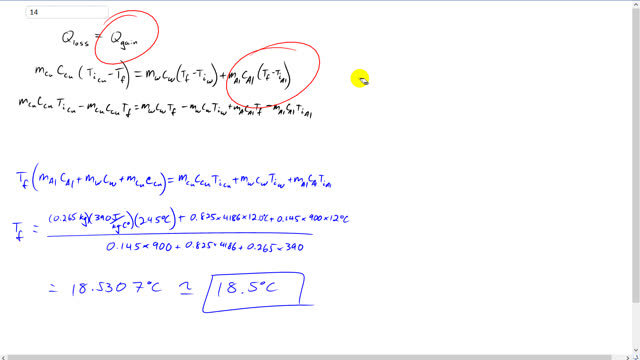## You are here

Question:

What will be the equilibrium temperature when a 265-g block of copper at $245 ^\circ \textrm{C}$ is placed in a 145-g aluminum calorimeter cup containing 825 g of water at $12.0 ^\circ \textrm{C}$?

Giancoli, Douglas C., Physics: Principles with Applications, 7th Ed., ©2014. Reprinted by permission of Pearson Education Inc., New York.
The question will be visible after logging in, as required by Pearson Education Inc.

$18.5^\circ\textrm{C}$
Note: At 2:53 I mis-spoke by saying 290J/kg instead of 390J/kg. It's written and calculated correctly.

# Giancoli 7th Edition, Chapter 14, Problem 14

(4:40)